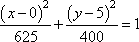Search

Conics: Ellipses: Word Problems (page 4 of 4)

Sections: Introduction, Finding information from the equation, Finding the equation from information, Word Problems• A "whispering room" is one with an elliptically-arched ceiling. If someone stands at one focus of the ellipse and whispers something to his friend, the dispersed sound waves are reflected by the ceiling and concentrated at the other focus, allowing people across the room to clearly hear what he said. Suppose such gallery has a ceiling reaching twenty feet above the five-foot-high vertical walls at its tallest point (so the cross-section is half an ellipse topping two vertical lines at either end), and suppose the foci of the ellipse are thirty feet apart. What is the height of the ceiling above each "whispering point"?
• Since the ceiling is half of an ellipse (the top half, specifically), and since the foci will be on a line between the tops of the "straight" parts of the side walls, the foci will be five feet above the floor, which sounds about right for people talking and listening: five feet high is close to face-high on most adults.

I'll center my ellipse above the origin, so (h, k) = (0, 5). The foci are thirty feet apart, so they're 15 units to either side of the center. In particular, c = 15. Since the elliptical part of the room's cross-section is twenty feet high above the center, and since this "shorter" direction is the semi-minor axis, then b = 20. The equation b2 = a2  c2 gives me 400 = a2  225, so a2 = 625. Then the equation for the elliptical ceiling is:I need to find the height of the ceiling above the foci. I prefer positive numbers, so I'll look at the focus to the right of the center. The height (from the ellipse's central line through its foci, up to the ceiling) will be the y-value of the ellipse when x = 15:144 + (y  5)2 = 400
(y  5)2 = 256

y  5 = ±16

y = 21

The ceiling is 21 feet above the floor.

• Satellites can be put into elliptical orbits if they need only sometimes to be in high- or low-earth orbit, thus avoiding the need for propulsion and navigation in low-earth orbit and the expense of launching into high-earth orbit. Suppose a satellite is in an elliptical orbit, with a = 4420 and b = 4416, and with the center of the Earth being at one of the foci of the ellipse. Assuming the Earth has a radius of about 3960 miles, find the lowest and highest altitudes of the satellite above the Earth.
• The lowest altitude will be at the vertex closer to the Earth; the highest altitude will be at the other vertex. Since I need to measure these altitudes from the focus, I need to find the value of c.

b2 = a2  c2
c2 = a2  b2 = 44202  44162 = 35,344

Then c = 188. If I set the center of my ellipse at the origin and make this a wider-than-tall ellipse, then I can put the Earth's center at the point (188, 0).

(This means, by the way, that there isn't much difference between the circumference of the Earth and the path of the satellite. The center of the elliptical orbit is actually inside the Earth, and the ellipse, having an eccentricity of e = 188 / 4420, or about 0.04, is pretty close to being a circle.)

The vertex closer to the end of the ellipse containing the Earth's center will be at 4420 units from the ellipse's center, or 4420  188 = 4232 units from the center of the Earth. Since the Earth's radius is 3960 units, then the altitude is 4232  3960 = 272. The other vertex is 4420 + 188 = 4608 units from the Earth's center, giving me an altitude of 4608  3960 = 648 units.

The minimum altitude is 272 miles above the Earth;
the maximum altitude is
648 miles above the Earth.

<< Previous  Top  |  1 | 2 | 3 | 4  |  Return to Index

 Cite this article as: Stapel, Elizabeth. "Conics: Ellipses: Word Problems." Purplemath. Available from     https://www.purplemath.com/modules/ellipse4.htm. Accessed [Date] [Month] 2016

Study Skills Survey

Tutoring from Purplemath
Find a local math tutor